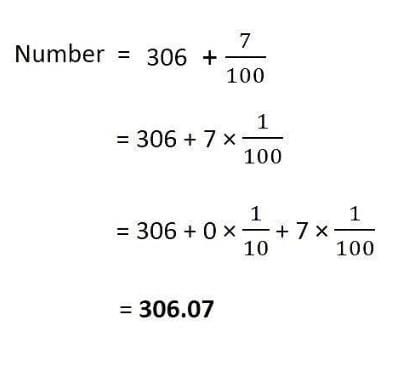Test: Decimals

# Test: Decimals

Test Description

## 10 Questions MCQ Test Mathematics (Maths) Class 6 | Test: Decimals

Test: Decimals for Class 6 2022 is part of Mathematics (Maths) Class 6 preparation. The Test: Decimals questions and answers have been prepared according to the Class 6 exam syllabus.The Test: Decimals MCQs are made for Class 6 2022 Exam. Find important definitions, questions, notes, meanings, examples, exercises, MCQs and online tests for Test: Decimals below.
Solutions of Test: Decimals questions in English are available as part of our Mathematics (Maths) Class 6 for Class 6 & Test: Decimals solutions in Hindi for Mathematics (Maths) Class 6 course. Download more important topics, notes, lectures and mock test series for Class 6 Exam by signing up for free. Attempt Test: Decimals | 10 questions in 10 minutes | Mock test for Class 6 preparation | Free important questions MCQ to study Mathematics (Maths) Class 6 for Class 6 Exam | Download free PDF with solutions
 1 Crore+ students have signed up on EduRev. Have you?
Test: Decimals - Question 1

### Write as decimal ‘Two ones and five-tenths’.

Test: Decimals - Question 2

### Decimal notation of 3/5 is _______.

Test: Decimals - Question 3

### Write 2 rupees and 5 paise as decimal.

Test: Decimals - Question 4

Decimal form of ‘seven-tenths’ is ________.

Detailed Solution for Test: Decimals - Question 4

The decimal 0.7 could be pronounced as SEVEN TENTHS or as ZERO POINT SEVEN.

Test: Decimals - Question 5

Decimal notation of one-tenth is ________.

Test: Decimals - Question 6

0.1 _____ 0.03

Detailed Solution for Test: Decimals - Question 6

The correct option is B as 0.1 is greater than 0.03.

Test: Decimals - Question 7

Decimal form of ‘Three hundred six and seven-hundredths’ is _______.

Detailed Solution for Test: Decimals - Question 7Test: Decimals - Question 8

What will be value of (8.5 x 5.8+ 8.5 x 4.2)/(1.7 x 7.6 - 1.7 x 6.6)?

Detailed Solution for Test: Decimals - Question 8

(8.5 x 5.8 + 8.5 x 4.2/1.7 x 7.6 - 1.7 x 6.6)

= [8.5 (5.8 + 4.2)/1.7(7.6 - 6.6)]

= (8.5 x 10/1.7 x 1)

= 85/1.7

= 50

Test: Decimals - Question 9

Every decimal can be written as a _________.

Test: Decimals - Question 10

What will be the value of  1 x 0.3 x 0.01 x 0.003?

## Mathematics (Maths) Class 6

167 videos|275 docs|43 tests
 Use Code STAYHOME200 and get INR 200 additional OFF Use Coupon Code
Information about Test: Decimals Page
In this test you can find the Exam questions for Test: Decimals solved & explained in the simplest way possible. Besides giving Questions and answers for Test: Decimals, EduRev gives you an ample number of Online tests for practice

## Mathematics (Maths) Class 6

167 videos|275 docs|43 tests